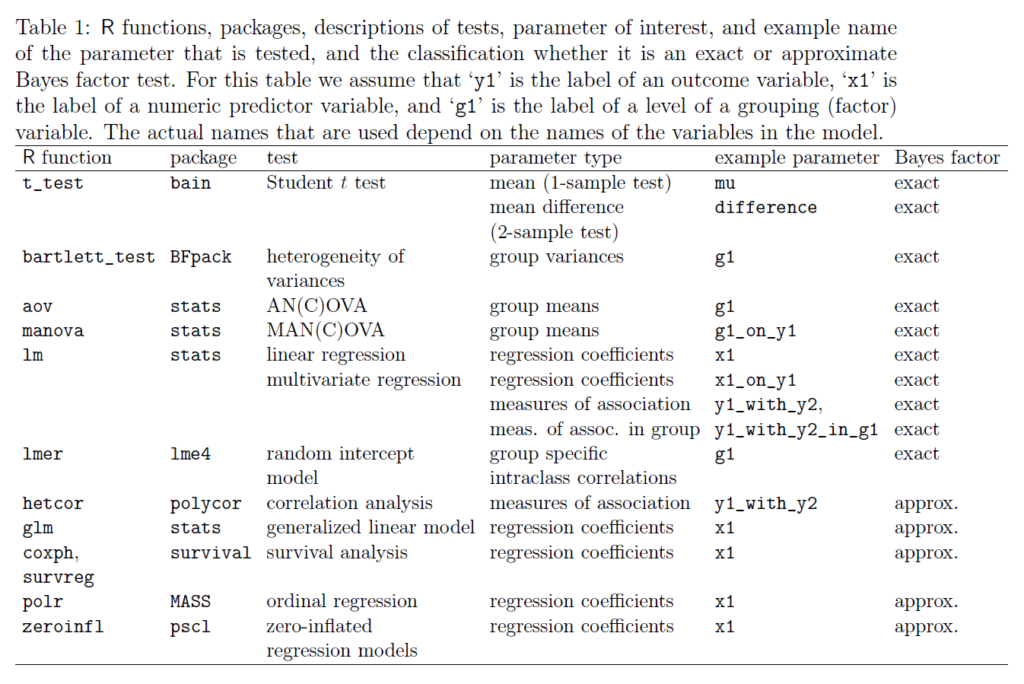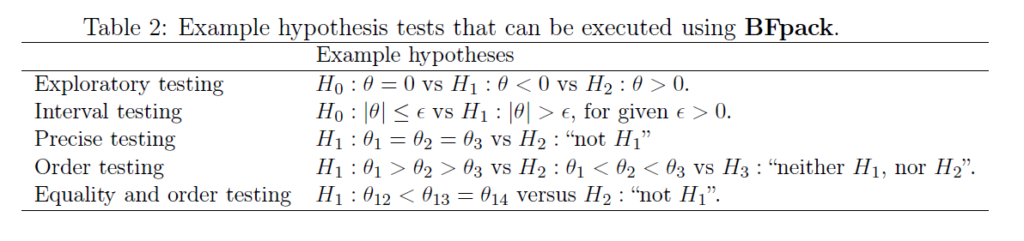# Preprint: BFpack — Flexible Bayes Factor Testing of Scientific Theories in R

This post is a synopsis of Mulder, J., Gu, X., Olsson-Collentine, A., Tomarken, A., Böing-Messing, F., Hoijtink, H., Meijerink, M., Williams, D. R., Menke, J., Fox, J.-P., Rosseel, Y., Wagenmakers, E.-J., & van Lissa, C. (2019). BFpack: Flexible Bayes factor testing of scientific theories in R. Preprint available at https://arxiv.org/pdf/1911.07728.pdf

## Abstract

“There has been a tremendous methodological development of Bayes factors for hypothesis testing in the social and behavioral sciences, and related fields. This development is due to the flexibility of the Bayes factor for testing multiple hypotheses simultaneously, the ability to test complex hypotheses involving equality as well as order constraints on the parameters of interest, and the interpretability of the outcome as the weight of evidence provided by the data in support of competing scientific theories. The available software tools for Bayesian hypothesis testing are still limited however. In this paper we present a new R-package called BFpack that contains functions for Bayes factor hypothesis testing for the many common testing problems. The software includes novel tools (i) for Bayesian exploratory testing (null vs positive vs negative effects), (ii) for Bayesian confirmatory testing (competing hypotheses with equality and/or order constraints), (iii) for common statistical analyses, such as linear regression, generalized linear models, (multivariate) analysis of (co)variance, correlation analysis, and random intercept models, (iv) using default priors, and (v) while allowing data to contain missing observations that are missing at random.”

## Overview of BFpack Functionality## A Variety of BFpack Test Questions## Example Applications

The preprint discusses seven application examples and illustrates each with R code. The examples concern (1) the t-test; (2) a 2-way ANOVA; (3) a test of equality of variances; (4) linear regression (with missing data) in fMRI research; (5) logistic regression in forensic psychology; (6) measures of association in neuropsychology; and (7) intraclass correlation.

### References

Mulder, J., Gu, X., Olsson-Collentine, A., Tomarken, A., Böing-Messing, F., Hoijtink, H., Meijerink, M., Williams, D. R., Menke, J., Fox, J.-P., Rosseel, Y., Wagenmakers, E.-J., & van Lissa, C. (2019). BFpack: Flexible Bayes factor testing of scientific theories in R. Preprint available at https://arxiv.org/pdf/1911.07728.pdf